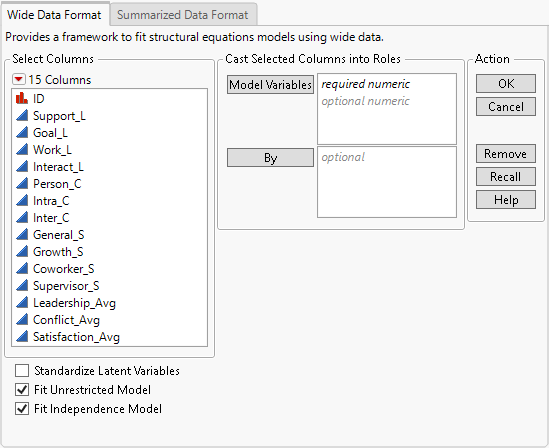Multivariate Methods > Structural Equation Models > Launch the Structural Equation Models Platform
Publication date: 05/24/2021

#Launch the Structural Equation Models Platform

Launch the Structural Equation Models platform by selecting Analyze > Multivariate Methods > Structural Equation Models.

Figure 8.4 Structural Equation Models Launch WindowThe Launch Window includes tabs for two different data formats.

Wide Data Format

Select for data tables where each row corresponds to a single observation and the columns contain variables to be used in the model. Rows that contain only missing values are excluded from the analysis.

Summarized Data Format

Select for data tables where the data are summarized as a correlation or covariance matrix. Means and standard deviations can also be specified as columns. If they are not specified, the means are assumed to be zero and the standard deviations are assumed to be the square root of the diagonal of the input matrix.

###Launch Window Options

Model Variables

The columns that you want to include in the model. You must specify at least one column. All columns must have numeric data type and continuous modeling type.

For the Summarized Data Format, the Model Variables columns are the columns that contain the correlation or covariance matrix for the summarized data.

By

(Available only for Wide Data Format.) A column that creates a report consisting of separate analyses for each level of the variable. If more than one By variable is assigned, a separate analysis is produced for each possible combination of the levels of the By variables.

Caution: Using a By variable does not perform a multiple-group analysis.

Mean

(Available only for Summarized Data Format.) A column of means that correspond to the variables in each row of the correlation or covariance matrix. If they are not specified, the means are assumed to be zero.

Std Dev

(Available only for Summarized Data Format.) A column of standard deviations that correspond to the variables in each row of the correlation or covariance matrix. If they are not specified, the standard deviations are assumed to be the square root of the diagonal of the input matrix.

Label

(Available only for Summarized Data Format.) A column of labels that correspond to the variables in each row of the correlation or covariance matrix. If they are not specified, the variables in the Model Specification use the names of the columns that contain the input matrix.

Standardize Latent Variables

If selected, this option sets the scale of the latent variables by fixing their variance to one and allowing free estimation of all loadings.

Fit Unrestricted Model

If selected, the unrestricted model is automatically fit when you fit your first model. You can then compare your fitted model to the unrestricted model in the Model Comparison report. The unrestricted model is a fully saturated model, which fits all means, variances, and covariances of the specified Model Variables without imposing any structure on the data.

Fit Independence Model

If selected, the independence model is automatically fit when you fit your first model. You can then compare your fitted model to the independence model in the Model Comparison report. The independence model fits all means and variances of the specified Model Variables. All covariances among the specified Model Variables are fixed to zero, which leads to a highly restrictive model.

Sample Size

(Available only for Summarized Data Format.) Specifies the number of observations represented by the summarized data.